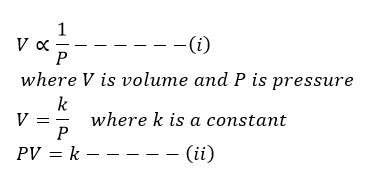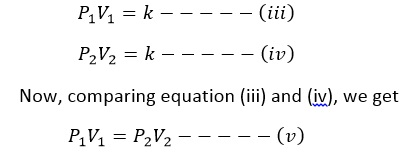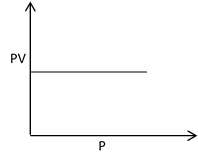# Boyle’s Law

Robert Boyle studied the variation of volume of given mass of gas with pressure at constant temperature. The result of his study in the form of law which is known as Boyle’s Law.
Boyle’s Law states. “The volume of a given mass of a gas is inversely proportional to its pressure at constant temperature.If P1 and V1 are initial pressure and volume and P2 and V2 are final pressure and volume, thenThus, using equation (v), we can determine the volume of given mass of gas at any pressure if we know its initial volume and pressure.
Note:
1 pascal = 1 N/m2
1 atm. = 760 mmHg = 1.01×105 N/m2
1 mmHg = 1 toor
1 toor = 105 N/m2

Experimental verification of Boyle’s Law:
The Boyle’s law is verified by plotting the graph between pressure against volume, volume against 1/pressure and pressure × volume against pressure.

The plot of pressure (P) against volume (V) at constant temperature gives a hyperbolic curve. At very low pressure volume of gas in high and as the pressure is increased, volume of gas goes on decreasing.

The plot of V against 1/P gives a straight line passing through origin. This also verifies Boyle’s law.Plot of PV against P gives a straight line parallel to X-axis.